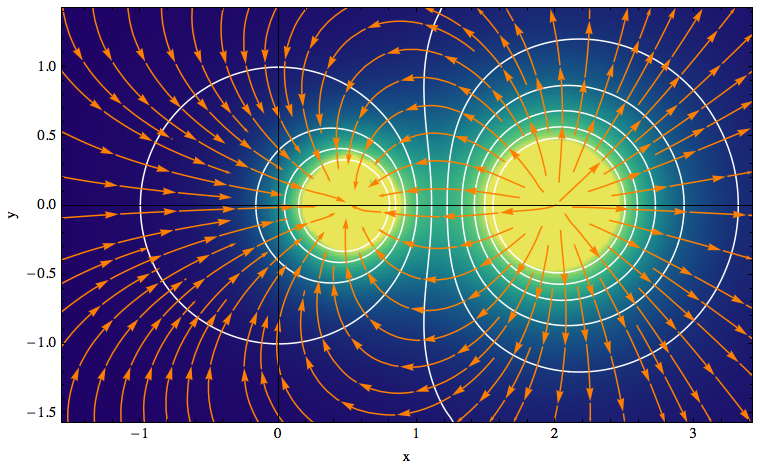This is the HTML version of a Mathematica 8/9 notebook. You can copy and paste the following into a notebook as literal plain text. For general notes and other related examples, see this page.

The function `listGradientFieldPlot` takes a scalar potential on a two-dimensional rectangular grid as its first argument, in the form of a list `φ`. The lengths corresponding to the grid dimensions can be given through the option `DataRange → {{xmin, xmax}, {ymin, ymax}}`.

The plot contains three elements:

• a contour plot of the potential `φ`,
• a colored density plot of the gradient field, `∇φ`,
• and a stream plot illustrating the field lines of `∇φ` (they are everywhere perpendicular to the contour lines of the potential).

Because the potential is given as a list, I could calculate the gradient either from an interpolating function or by using discrete first-order derivatives. In fact, the built-in function `DerivativeFilter` can be used to calculate directional derivatives of arrays, and it uses interpolation. Some interesting uses of this filter are shown in this blog entry.

Unfortunately, interpolation can introduce artifacts. For example, define a rapidly varying function on a grid by
`m = Table[(y^2 + (x - 2)^2)^(-1/2), {x, -1.57, 3.43, .1}, {y, -1.57, 1.43, .1}];`
and then execute the test cases
`Table[Graphics@Raster[Abs@DerivativeFilter[m, {1, 0}, InterpolationOrder -> i]], {i, 3, 9, 2}]`
This will reveal an increasing number of undesirable ripples. Moreover, `DerivativeFilter` doesn't allow the padding option `"Extrapolated"` which is needed to get reasonable derivatives at the boundary of a region.

Therefore, to do the gradient of an array, I decided to calculate derivatives without interpolation by means of a standard finite-difference scheme. This is done in the variables `delX` and `delY` below, using an auxiliary array that has been padded at the borders using extrapolation.

```listGradientFieldPlot[grid_?((Length[Dimensions[#]] == 2) &),
opts : OptionsPattern[]] :=
Module[
{
img,
cont,
densityOptions,
contourOptions,
frameOptions,
plotRangeRule,
delX,
delY,
gridSpacing,
gradField,
gradNorm,
field,
fieldL,
rangeCoords,
maxNorm,
paddedGrid = ArrayPad[grid, 1, "Extrapolated"]
},
gridSpacing = (DataRange /. {opts}).{-1, 1};
If[! NumericQ[Norm[gridSpacing]], gridSpacing = {1, 1},
gridSpacing = gridSpacing/Reverse[Dimensions[grid] - 1]];
densityOptions = Join[
FilterRules[{opts},
FilterRules[Options[ListDensityPlot],
Except[{Prolog, Epilog, FrameTicks, PlotLabel, ImagePadding, GridLines, Mesh, AspectRatio, PlotLabel,
PlotRangePadding, Frame, Axes}]]],
{PlotRangePadding -> None, Frame -> None, Axes -> None,
AspectRatio -> Automatic}
];
contourOptions = Join[
FilterRules[{opts},
FilterRules[Options[ListContourPlot],
Except[{Prolog, Epilog, FrameTicks, PlotLabel, Background, ContourShading, Frame,
Axes}]]],
{Frame -> None, Axes -> None, ContourShading -> False}
];
delX = (RotateRight[paddedGrid, {0, 1}] -
RotateLeft[paddedGrid, {0, 1}])[[2 ;; -2, 2 ;; -2]]/
gridSpacing[];
delY = (RotateRight[paddedGrid] - RotateLeft[paddedGrid])[[2 ;; -2,
2 ;; -2]]/gridSpacing[];
gradNorm = Sqrt[delX*delX + delY*delY];
gradField =
MapThread[{#2, #1} &, {Transpose[delY], Transpose[delX]}, 2];
maxNorm = Max[Abs[gradNorm]];
gradField = Chop[gradField/maxNorm];
fieldL =
ListDensityPlot[gradNorm, Evaluate@Apply[Sequence, densityOptions]];
field=First@Cases[{fieldL},Graphics[__],\[Infinity]];
plotRangeRule = FilterRules[Quiet@AbsoluteOptions[field], PlotRange];
rangeCoords = Transpose[PlotRange /. plotRangeRule];
img = Rasterize[field, "Image"];
cont = If[
MemberQ[{0, None}, (Contours /. FilterRules[{opts}, Contours])],
{},
ListContourPlot[grid,
Evaluate@Apply[Sequence, contourOptions]]];
frameOptions = Join[
FilterRules[{opts},
FilterRules[Options[Graphics],
Except[{PlotRangeClipping, PlotRange}]]],
{plotRangeRule, Frame -> True, PlotRangeClipping -> True, PlotLabel -> Row[{"Maximum field =", maxNorm}]}
];
If[Head[fieldL]===Legended,Legended[#,fieldL[]],#]&@
Apply[Show[
Graphics[
{
Inset[
Show[SetAlphaChannel[img,
"ShadingOpacity" /. {opts} /. {"ShadingOpacity" -> 1}],
AspectRatio -> Full], rangeCoords[], {0, 0},
rangeCoords[] - rangeCoords[]]
}],
cont,
ListStreamPlot[gradField,
Evaluate@FilterRules[{opts}, StreamStyle],
Evaluate@FilterRules[{opts}, StreamColorFunction],
Evaluate@FilterRules[{opts}, DataRange],
Evaluate@FilterRules[{opts}, StreamColorFunctionScaling],
Evaluate@FilterRules[{opts}, StreamPoints],
Evaluate@FilterRules[{opts}, StreamScale]],
##
] &, frameOptions]
]
```

Here is an example of how to use the function. The contour lines of the potential are shown in white, and the streamlines of the gradient field are orange.

```grid = Transpose@
Table[(y^2 + (x - 2)^2)^(-1/2) - (y^2 + (x - 1/2)^2)^(-1/2)/
2, {x, -1.57, 3.43, .1}, {y, -1.57, 1.43, .1}];

l1 = listGradientFieldPlot[grid, ColorFunction -> "BlueGreenYellow",
Contours -> 10, ContourStyle -> White, Frame -> True,
FrameLabel -> {"x", "y"}, InterpolationOrder -> 2,
ClippingStyle -> Automatic, Axes -> True, StreamStyle -> Orange,
ImageSize -> 500, DataRange -> {{-1.57, 3.43}, {-1.57, 1.43}}]
```noeckel@uoregon.edu
Last modified: Tue Aug 19 11:45:48 PDT 2014